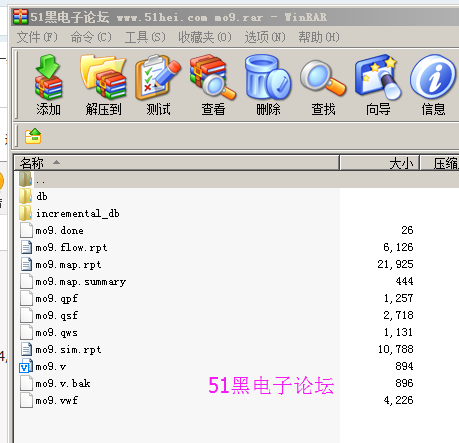##帐号 自动登录 找回密码 密码 立即注册

# Verilog设计一异步复位、模9，带进位端的计数器，用状态机实现ID:209315 发表于 2017-6-11 11:47 | 显示全部楼层 |阅读模式module mo9(clk,clr,z,out); input clk,clr; output reg z; output reg [3:0] out; parameter  s0=0,s1=1,s2=2, s3=3,s4=4, s5=5,s6=6,s7=7,s8=8; reg [3:0]  next_state,state; always @(posedge clk or posedge clr) begin               if(clr)         state<=s0;         else                 state<=next_state; end always @(state) begin case (state)                 s0: begin next_state<=s1; out<=state;end                 s1: begin next_state<=s2; out<=state;end                 s2: begin next_state<=s3; out<=state;end                 s3: begin next_state<=s4; out<=state;end                 s4: begin next_state<=s5; out<=state;end                 s5: begin next_state<=s6; out<=state;end                 s6: begin next_state<=s7; out<=state;end                 s7: begin next_state<=s8; out<=state;end                 s8: begin next_state<=s0; out<=state;end                 default: begin next_state<=s0; out<=state;end                 endcase                 end always @(state) begin           case(state)                 s8: z<=1'b1;                 default:z<=1'b0;          endcase end endmodule 复制代码 全部资料下载地址：mo9.rar (98.81 KB, 下载次数: 8) 2017-6-11 11:47 上传 点击文件名下载附件

### 评分

 本版积分规则 回帖后跳转到最后一页